Q-Chem 5.0 User’s Manual

# 4.12 Unconventional SCF Calculations

## 4.12.1 Polarized Atomic Orbital (PAO) Calculations

Polarized atomic orbital (PAO) calculations are an interesting unconventional SCF method, in which the molecular orbitals and the density matrix are not expanded directly in terms of the basis of atomic orbitals. Instead, an intermediate molecule-optimized minimal basis of polarized atomic orbitals (PAOs) is used . The polarized atomic orbitals are defined by an atom-blocked linear transformation from the fixed atomic orbital basis, where the coefficients of the transformation are optimized to minimize the energy, at the same time as the density matrix is obtained in the PAO representation. Thus a PAO-SCF calculation is a constrained variational method, whose energy is above that of a full SCF calculation in the same basis. However, a molecule optimized minimal basis is a very compact and useful representation for purposes of chemical analysis, and it also has potential computational advantages in the context of MP2 or local MP2 calculations, as can be done after a PAO-HF calculation is complete to obtain the PAO-MP2 energy.

PAO-SCF calculations tend to systematically underestimate binding energies (since by definition the exact result is obtained for atoms, but not for molecules). In tests on the G2 database, PAO-B3LYP/6-311+G(2df,p) atomization energies deviated from full B3LYP/6-311+G(2df,p) atomization energies by roughly 20 kcal/mol, with the error being essentially extensive with the number of bonds. This deviation can be reduced to only 0.5 kcal/mol with the use of a simple non-iterative second order correction for “beyond-minimal basis” effects . The second order correction is evaluated at the end of each PAO-SCF calculation, as it involves negligible computational cost. Analytical gradients are available using PAOs, to permit structure optimization. For additional discussion of the PAO-SCF method and its uses, see the references cited above.

Calculations with PAOs are determined controlled by the following \$rem variables. PAO_METHOD = PAO invokes PAO-SCF calculations, while the algorithm used to iterate the PAOs can be controlled with PAO_ALGORITHM.

PAO_ALGORITHM
 Algorithm used to optimize polarized atomic orbitals (see PAO_METHOD)

TYPE:
 INTEGER

DEFAULT:
 0

OPTIONS:
 0 Use efficient (and riskier) strategy to converge PAOs. 1 Use conservative (and slower) strategy to converge PAOs.

RECOMMENDATION:
 None

PAO_METHOD
 Controls evaluation of polarized atomic orbitals (PAOs).

TYPE:
 STRING

DEFAULT:
 EPAO For local MP2 calculations Otherwise no default.

OPTIONS:
 PAO Perform PAO-SCF instead of conventional SCF. EPAO Obtain EPAOs after a conventional SCF.

RECOMMENDATION:
 None

## 4.12.2 SCF Meta-Dynamics

As the SCF equations are non-linear in the electron density, there are in theory very many solutions (i.e., sets of orbitals where the energy is stationary with respect to changes in the orbital subset). Most often sought is the solution with globally minimal energy as this is a variational upper bound to the true eigenfunction in this basis. The SCF methods available in Q-Chem allow the user to converge upon an SCF solution, and (using STABILITY_ANALYSIS) ensure it is a minimum, but there is no known method of ensuring that the found solution is a global minimum; indeed in systems with many low-lying energy levels the solution converged upon may vary considerably with initial guess.

SCF meta-dynamics  is a technique which can be used to locate multiple SCF solutions, and thus gain some confidence that the calculation has converged upon the global minimum. It works by searching out a solution to the SCF equations. Once found, the solution is stored, and a biasing potential added so as to avoid re-converging to the same solution. More formally, the distance between two solutions,and, can be expressed as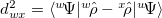, where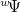is a Slater determinant formed from the orthonormal orbitals,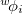, of solution, and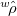is the one-particle density operator for. This definition is equivalent to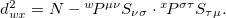and is easily calculated.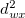is bounded by 0 and the number of electrons, and can be taken as the distance between two solutions. As an example, any singly excited determinant from an SCF determinant (which will not in general be another SCF solution), would be a distance 1 away from it.

In a manner analogous to classical meta-dynamics, to bias against the set of previously located solutions,, we create a new Lagrangian,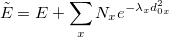(4.114)

where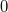represents the present density. From this we may derive a new effective Fock matrix,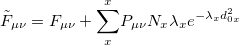(4.115)

This may be used with very little modification within a standard DIIS procedure to locate multiple solutions. When close to a new solution, the biasing potential is removed so the location of that solution is not affected by it. If the calculation ends up re-converging to the same solution,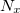and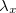can be modified to avert this. Once a solution is found it is added to the list of solutions, and the orbitals mixed to provide a new guess for locating a different solution.

This process can be customized by the REM variables below. Both DIIS and GDM methods can be used, but it is advisable to turn on MOM when using DIIS to maintain the orbital ordering. Post-HF correlation methods can also be applied. By default they will operate for the last solution located, but this can be changed with the SCF_MINFIND_RUNCORR variable.

The solutions found through meta-dynamics also appear to be good approximations to diabatic surfaces where the electronic structure does not significantly change with geometry. In situations where there are such multiple electronic states close in energy, an adiabatic state may be produced by diagonalizing a matrix of these states - Configuration Interaction. As they are distinct solutions of the SCF equations, these states are non-orthogonal (one cannot be constructed as a single determinant made out of the orbitals of another), and so the CI is a little more complicated and is a non-orthogonal CI (NOCI). More information on NOCI can be found in Section 6.2.6.

SCF_SAVEMINIMA
 Turn on SCF meta-dynamics and specify how many solutions to locate.

TYPE:
 INTEGER

DEFAULT:
 0

OPTIONS:
 0 Do not use SCF meta-dynamicsAttempt to finddistinct SCF solutions.

RECOMMENDATION:
 Perform SCF Orbital meta-dynamics and attempt to locatedifferent SCF solutions. Note that these may not all be minima. Many saddle points are often located. The last one located will be the one used in any post-SCF treatments. In systems where there are infinite point groups, this procedure cannot currently distinguish between spatial rotations of different densities, so will likely converge on these multiply.

 Read in solutions from a previous SCF meta-dynamics calculation

TYPE:
 INTEGER

DEFAULT:
 0

OPTIONS:Read inprevious solutions and attempt to locate them all.Read inprevious solutions, but only attempt to locate solution.

RECOMMENDATION:
 This may not actually locate all solutions required and will probably locate others too. The SCF will also stop when the number of solutions specified in SCF_SAVEMINIMA are found. Solutions from other geometries may also be read in and used as starting orbitals. If a solution is found and matches one that is read in within SCF_MINFIND_READDISTTHRESH, its orbitals are saved in that position for any future calculations. The algorithm works by restarting from the orbitals and density of a the minimum it is attempting to find. After 10 failed restarts (defined by SCF_MINFIND_RESTARTSTEPS), it moves to another previous minimum and attempts to locate that instead. If there are no minima to find, the restart does random mixing (with 10 times the normal random mixing parameter).

SCF_MINFIND_WELLTHRESH
 Specify what SCF_MINFIND believes is the basin of a solution

TYPE:
 INTEGER

DEFAULT:
 5

OPTIONS:for a threshold of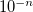RECOMMENDATION:
 When the DIIS error is less than, penalties are switched off to see whether it has converged to a new solution.

SCF_MINFIND_RESTARTSTEPS
 Restart with new orbitals if no minima have been found within this many steps

TYPE:
 INTEGER

DEFAULT:
 300

OPTIONS:Restart aftersteps.

RECOMMENDATION:
 If the SCF calculation spends many steps not finding a solution, lowering this number may speed up solution-finding. If the system converges to solutions very slowly, then this number may need to be raised.

SCF_MINFIND_INCREASEFACTOR
 Controls how the height of the penalty function changes when repeatedly trapped at the same solution

TYPE:
 INTEGER

DEFAULT:
 10100 meaning 1.01

OPTIONS: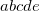corresponding to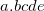RECOMMENDATION:
 If the algorithm converges to a solution which corresponds to a previously located solution, increase both the normalization N and the width lambda of the penalty function there. Then do a restart.

SCF_MINFIND_INITLAMBDA
 Control the initial width of the penalty function.

TYPE:
 INTEGER

DEFAULT:
 02000 meaning 2.000

OPTIONS:corresponding to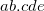RECOMMENDATION:
 The initial inverse-width (i.e., the inverse-variance) of the Gaussian to place to fill solution’s well. Measured in electrons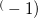. Increasing this will repeatedly converging on the same solution.

SCF_MINFIND_INITNORM
 Control the initial height of the penalty function.

TYPE:
 INTEGER

DEFAULT:
 01000 meaning 1.000

OPTIONS:corresponding toRECOMMENDATION:
 The initial normalization of the Gaussian to place to fill a well. Measured in hartrees.

SCF_MINFIND_RANDOMMIXING
 Control how to choose new orbitals after locating a solution

TYPE:
 INTEGER

DEFAULT:

OPTIONS:corresponding toradians

RECOMMENDATION:
 After locating an SCF solution, the orbitals are mixed randomly to move to a new position in orbital space. For each occupied and virtual orbital pair picked at random and rotate between them by a random angle between 0 and this. If this is negative then use exactly this number, e.g.,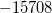will almost exactly swap orbitals. Any number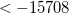will cause the orbitals to be swapped exactly.

SCF_MINFIND_NRANDOMMIXES
 Control how many random mixes to do to generate new orbitals

TYPE:
 INTEGER

DEFAULT:
 10

OPTIONS:Performrandom mixes.

RECOMMENDATION:
 This is the number of occupied/virtual pairs to attempt to mix, per separate density (i.e., for unrestricted calculations both alpha and beta space will get this many rotations). If this is negative then only mix the highest 25% occupied and lowest 25% virtuals.

 The distance threshold at which to consider two solutions the same

TYPE:
 INTEGER

DEFAULT:
 00100 meaning 0.1

OPTIONS:corresponding toRECOMMENDATION:
 The threshold to regard a minimum as the same as a read in minimum. Measured in electrons. If two minima are closer together than this, reduce the threshold to distinguish them.

SCF_MINFIND_MIXMETHOD
 Specify how to select orbitals for random mixing

TYPE:
 INTEGER

DEFAULT:
 0

OPTIONS:
 0 Random mixing: select from any orbital to any orbital. 1 Active mixing: select based on energy, decaying with distance from the Fermi level. 2 Active Alpha space mixing: select based on energy, decaying with distance from the Fermi level only in the alpha space.

RECOMMENDATION:
 Random mixing will often find very high energy solutions. If lower energy solutions are desired, use 1 or 2.

SCF_MINFIND_MIXENERGY
 Specify the active energy range when doing Active mixing

TYPE:
 INTEGER

DEFAULT:
 00200 meaning 00.200

OPTIONS:corresponding toRECOMMENDATION:
 The standard deviation of the Gaussian distribution used to select the orbitals for mixing (centered on the Fermi level). Measured in Hartree. To find less-excited solutions, decrease this value

SCF_MINFIND_RUNCORR
 Run post-SCF correlated methods on multiple SCF solutions

TYPE:
 INTEGER

DEFAULT:
 0

OPTIONS:
 If this is set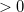, then run correlation methods for all found SCF solutions.

RECOMMENDATION:
 Post-HF correlation methods should function correctly with excited SCF solutions, but their convergence is often much more difficult owing to intruder states.